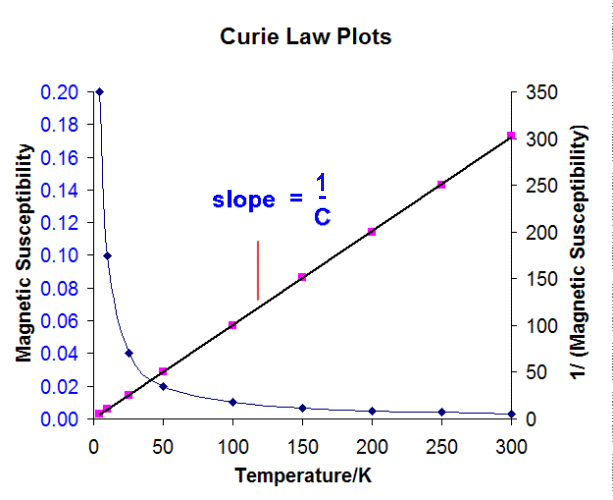20.10C: The Effects of Temperature on Magnetic Moment

Normal paramagnetic substances obey the Curie Law

$\chi = \dfrac{C}{T}$

where $$C$$ is the Curie constant. Thus a plot of 1/ χ versus $$T$$ should give a straight line of slope 1/C passing through the origin (0 K). Whereas many substances do give a straight line it often intercepts just a little above 0 K and these are said to obey the Curie-Weiss Law:

$\chi =\dfrac{C}{T+Φ}$

where $$Φ$$ is known as the Weiss constant.Quantum Mechanics Approach

A similar expression (where χ is inversely proportional to Temperature) is obtained but now the constant $$C$$ is given by the Langevin expression, which relates the susceptibility to the magnetic moment:

$\chi_m =\dfrac{N \mu^2}{3kT}$

where

• $$N$$ is Avogadro's number
• $$k$$ is the Boltzmann constant and
• $$T$$ the absolute temperature

rewriting this gives the magnetic moment as

$μ= 2.828 \sqrt{\chi_mT} B.M.$

There are two main types of magnetic compounds, those that are diamagnetic (compounds that are repelled by a magnetic field) and those that are paramagnetic (compounds that are attracted by a magnetic field). All substances possess the property of diamagnetism due to the presence of closed shells of electrons within the substance. Note that diamagnetism is a weak effect while paramagnetism is a much stronger effect.

Paramagnetism derives from the spin and orbital angular momenta of electrons. This type of magnetism occurs only in compounds containing unpaired electrons, as the spin and orbital angular momenta is canceled out when the electrons exist in pairs. Compounds in which the paramagnetic centers are separated by diamagnetic atoms within the sample are said to be magnetically dilute.

If the diamagnetic atoms are removed from the system then the paramagnetic centers interact with each other. This interaction leads to ferromagnetism (in the case where the neighboring magnetic dipoles are aligned in the same direction) and antiferromagnetism (where the neighboring magnetic dipoles are aligned in alternate directions).

These two forms of paramagnetism show characteristic variations of the magnetic susceptibility with temperature.In the case of ferromagnetism, above the Curie point the material displays "normal" paramagnetic behavior. Below the Curie point the material displays strong magnetic properties.

• Ferromagnetism is commonly found in compounds containing iron and in alloys.
• For antiferromagnetism, above the Neel point the material displays "normal" paramagnetic behavior. Below the Neel point the material displays weak magnetic properties which at lower and lower temperatures can become essentially diamagnetic. Antiferromagnetism is more common and is found to occur in transition metal halides and oxides, such as TiCl3 and VCl2.